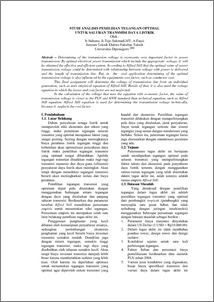# STUDI ANALISIS PEMILIHAN TEGANGAN OPTIMAL UNTUK SALURAN TRANSMISI DAYA LISTRIK

Fauzi, Akhmad and Sulasno, Sulasno and Sukmadi, Tedjo (2011) STUDI ANALISIS PEMILIHAN TEGANGAN OPTIMAL UNTUK SALURAN TRANSMISI DAYA LISTRIK. Undergraduate thesis, Jurusan Teknik Elektro Fakultas Teknik Undip.Preview
PDF - Published Version
375Kb

## Abstract

Determining of the transmission voltage is represents very important factor in power transmission. By optimal electrical power transmission which include the appropriate voltage, it will be obtained the effective and efficient system. According to Alfred Still that the optimal value of power transmission voltage could be determined with relationship between voltage with power is delivered and the length of transmission line. But, in the real application determining of the optimal transmission voltage is also influenced by the equipments cost factor, such as conductor cost. This final assignment will determine the voltage of transmission line from an individual generation, such as uses empirical equation of Alfred Still. Beside of that, it is also used the voltage equation in which the losses and cost factor are not neglected. In the calculation of the voltage that uses the equation with economic factor, the value of transmission voltage is closer to the PLN and ANSI standard than technical equation, such as Alfred Still equation. Alfred Still equation is used for determining the transmission voltage technically, because it neglects the cost factor.

Item Type: Thesis (Undergraduate) T Technology > TK Electrical engineering. Electronics Nuclear engineering Faculty of Engineering > Department of Electrical EngineeringFaculty of Engineering > Department of Electrical Engineering 25279 Mr. Sudjadi Pranoto 10 Jan 2011 16:54 10 Jan 2011 16:54

Repository Staff Only: item control page